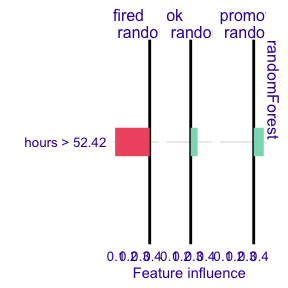# Explaining classification models with localModel package

Explaining classification models with the localModel package is just as simple as explaining regression. It is enough to work with predicted scores (class probabilities) rather than classes. In multiclass setting, a separate explanation is provided for each class probability.

We will work with the HR dataset from DALEX2 package. As in the regression example from Introduction to the localModel package, we will first create a random forest model and a DALEX2 explainer. Details about the method can be found in the Methodology behind localModel package vignette.

library(DALEX)
library(randomForest)
library(localModel)

data('HR')

set.seed(17)
mrf <- randomForest(status ~., data = HR, ntree = 100)
explainer <- explain(mrf,
HR[, -6],
predict_function = function(x, y) predict(x, y, type = "prob"))
#> Preparation of a new explainer is initiated
#>   -> model label       :  randomForest  ( [33m default [39m )
#>   -> data              :  7847  rows  5  cols
#>   -> target variable   :  not specified! ( [31m WARNING [39m )
#>   -> predict function  :  function(x, y) predict(x, y, type = "prob")
#>   -> predicted values  :  No value for predict function target column. ( [33m default [39m )
#>   -> model_info        :  package randomForest , ver. 4.6.14 , task multiclass ( [33m default [39m )
#>   -> model_info        :  Model info detected multiclass task but 'y' is a NULL .  ( [31m WARNING [39m )
#>   -> model_info        :  By deafult multiclass tasks supports only factor 'y' parameter.
#>   -> model_info        :  Consider changing to a factor vector with true class names.
#>   -> model_info        :  Otherwise I will not be able to calculate residuals or loss function.
#>   -> predicted values  :  predict function returns multiple columns:  3  ( [33m default [39m )
#>   -> residual function :  difference between 1 and probability of true class ( [33m default [39m )
#>  [32m A new explainer has been created! [39m
new_observation <- HR[10, -6]
new_observation
#>    gender      age    hours evaluation salary
#> 12 female 33.16119 55.08747          4      4

In DALEX2, we have built-in predict functions for some types of models. Random Forest is among these models.

model_lok <- individual_surrogate_model(explainer, new_observation,
size = 500, seed = 17)
plot(model_lok)plot(model_lok, geom = "bar")The plot shows how predictions for different classes are influenced by different features. For the actually predicted class, hours and evaluation have a strong positive effect.